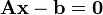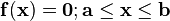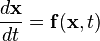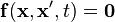# Category:Solvers

(Redirected from Solvers)
NLA
QRSlv
CMSlv
IPSlv
NLP
CONOPT
IPOPT
TRON
MINOS
Opt
NGSlv
DAE/ODE
IDA
LSODE
DOPRI5
LA
Linsolqr
Linsol
LP
MakeMPS
Logic
LRSlv

This is the complete list of solvers provided by ASCEND. At right, the status of solvers (working or broken or dead) is shown by colour.

The types of solver available in ASCEND include

• LA linear algebraic solvers$\mathbf A \mathbf x - \mathbf b = \mathbf 0$
• NLA non-linear algebraic solvers$\mathbf f(\mathbf x) = \mathbf 0; \mathbf a \le \mathbf x \le \mathbf b$
• ODE ordinary differential equation solvers$\frac{d \mathbf x}{d t} = \mathbf f(\mathbf x,t)$
• DAE differential-algebraic equation solvers$\mathbf f(\mathbf x,\mathbf x',t) = \mathbf 0$
• NLP non-linear programming solvers (optimisation)

Solvers of all of these types exist and are currently useful. Some API design issues still need work, including the way in which ASCEND implements derivatives in equations.

Currently all the NLA/NLP solvers operate via the 'SLV' API, the ODE and DAE solvers use the 'Integrator' API, and the LA solver have sort-of their own linear API (although they are effectively different).

## Subcategories

This category has the following 3 subcategories, out of 3 total.

## Pages in category "Solvers"

The following 24 pages are in this category, out of 24 total.